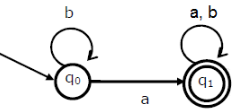Courses

# Test: Regular Expressions & Finite Automata- 1

## 20 Questions MCQ Test GATE Computer Science Engineering(CSE) 2022 Mock Test Series | Test: Regular Expressions & Finite Automata- 1

Description
This mock test of Test: Regular Expressions & Finite Automata- 1 for Computer Science Engineering (CSE) helps you for every Computer Science Engineering (CSE) entrance exam. This contains 20 Multiple Choice Questions for Computer Science Engineering (CSE) Test: Regular Expressions & Finite Automata- 1 (mcq) to study with solutions a complete question bank. The solved questions answers in this Test: Regular Expressions & Finite Automata- 1 quiz give you a good mix of easy questions and tough questions. Computer Science Engineering (CSE) students definitely take this Test: Regular Expressions & Finite Automata- 1 exercise for a better result in the exam. You can find other Test: Regular Expressions & Finite Automata- 1 extra questions, long questions & short questions for Computer Science Engineering (CSE) on EduRev as well by searching above.
QUESTION: 1

### Consider the languages L1 =and L2 = {a}. Which one of the following represents L1 L2* U L1*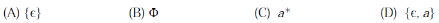Solution:
• Since L1 is empty.The concatenation of any other language with empty language is always empty.
• Hence L1 L2*  evaluates to be empty language. Hence , L1 L2* = {Φ} .
• a* = {Φ} The kleene closure of empty language always gives null string hence  Φ* = {∈}
• Final answer evaluates to be  {Φ} U {∈} = {∈}.
QUESTION: 2

### Consider the DFA given.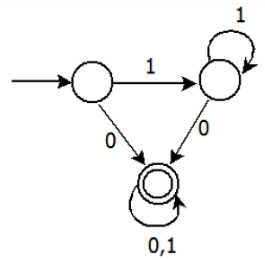Which of the following are FALSE? 1. Complement of L(A) is context-free. 2. L(A) = L((11*0+0)(0 + 1)*0*1*) 3. For the language accepted by A, A is the minimal DFA. 4. A accepts all strings over {0, 1} of length at least 2.

Solution:

Explanation :

(1) L(A) is regular, its complement is also regular and if it is regular it is also context free.
(2) L ( A ) = ( 11*0 + 0 )( 0 + 1 ) * 0*1* = 1*0 ( 0 + 1 ) *  Language has all strings where each string contains ‘0’.
(3) A is not minimal, it can be constructed with 2 states
(4) Language has all strings, where each string contains ‘0’. ( atleast length one)

QUESTION: 3

### W hat is the complement of the language accepted by the NFA shown below?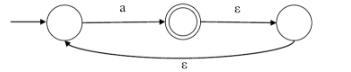Solution:

The given alphabet contains only one symbol {a} and the given NFA accepts all strings with any number of occurrences of ‘a’. In other words, the NFA accepts a+. Therefore complement of the language accepted by automata is empty string.

QUESTION: 4

Given the language, L = {ab, aa, baa}, which of the following strings are in L*?

1. abaabaaabaa
2. aaaabaaaa
3. baaaaabaaaab
4. baaaaabaa

Solution:
1. Any combination of strings in set {ab, aa, baa} will be in L*.….1) 1. “abaabaaabaa” can be partitioned as a combination of strings in set {ab, aa, baa}. The partitions are “ab aa baa ab aa".
2. “aaaabaaaa” can be partitioned as a combination of strings in set {ab, aa, baa}. The partitions are “aa ab aa aa”
3.  “baaaaabaaaab” cannot be partitioned as a combination of strings in set {ab, aa, baa}.
4.  “baaaaabaa” can be partitioned as a combination of strings in set {ab, aa, baa}. The partitions are “baa aa ab aa”

Hence, the correct answer is option C.

QUESTION: 5

Consider the set of strings on {0,1} in which, every substring of 3 symbols has at most two zeros. For example, 001110 and 011001 are in the language, but 100010 is not. All strings of length less than 3 are also in the language. A partially completed DFA that accepts this language is shown below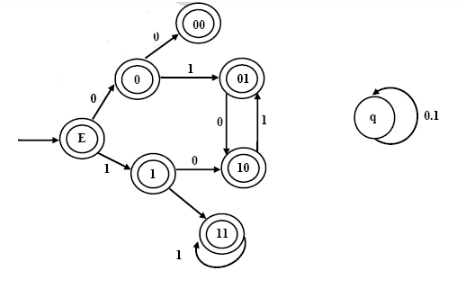Solution:

'000' cannot be accepted. So, '00' when gets another 0 must go to a dead state, q.

So, options A and B are eliminated.

By analyzing options C and D, we can understand that when states like 00, 01, 11 or 10 get an input 1 or 0, the next state is the last two digits of the newly formed string.

11 +0 -> 110 (So, 11 on 0 goes to 10).

01+1 -> 011 (So, 01 on 1 goes to 11).

So option D is correct.

QUESTION: 6

Definition of a language L with alphabet {a} is given as following.

L={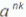| k>0, and n is a positive integer constant}

Q. What is the minimum number of states needed in DFA to recognize L?

Solution: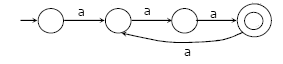For n=3 and k=1,2,3,4,....

it a^3,a^6....so n+1 state

Since n is constant and k is changing but logic is k>0

So n+1 required.

QUESTION: 7

A deterministic finite automation (DFA)D with alphabet {a,b} is given below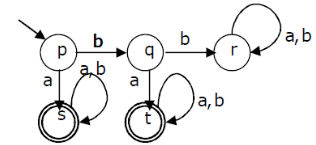Which of the following finite state machines is a valid minimal DFA which accepts the same language as D?

Solution:

Options (B) and (C) are invalid because they both accept ‘b’ as a string which is not accepted by give DFA. (D) is invalid because it accepts "bba" which are not accepted by given DFA.

QUESTION: 8

Let w be any string of length n is {0,1}*. Let L be the set of all substrings of w. What is the minimum number of states in a non-deterministic finite automaton that accepts L?

Solution:

We need minimum n+1 states to build NFA that accepts all substrings of a binary string. For example, following NFA accepts all substrings of “010″ and it has 4 states.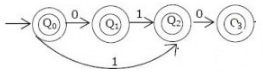QUESTION: 9

Which one of the following languages over the alphabet {0,1} is described by the regular expression: (0+1)*0(0+1)*0(0+1)*?

Solution:

The regular expression has two 0′s surrounded by (0+1)* which means accepted strings must have at least 2 0′s.

QUESTION: 10

Which one of the following is FALSE?

Solution:

Deterministic PDA cannot handle languages or grammars with ambiguity, but NDPDA can handle languages with ambiguity and any context-free grammar. So every nondeterministic PDA can not be converted to an equivalent deterministic PDA.

QUESTION: 11

Given the following state table of an FSM with two states A and B, one input and one output: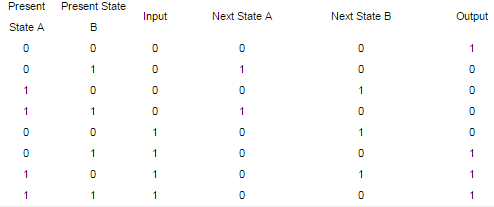Q. If the initial state is A=0, B=0, what is the minimum length of an input string which will take the machine to the state A=0, B=1 with Output = 1?

Solution:

//(0, 0) --1--> (0, 1) --0-->(1, 0) --1--> (0, 1) and output 1 According to the question we have to reach the states A=0, B=1 and output=1. This state is shown by green color. Thus to reach final states as A=0, B=1 and output=1 we have to reach previous states of A=1, B=0. Since initial states are A=0,B=0 (red); we provide input=1 (to reach A=0,B=1) Now this will give present states as A=0, B=1 and output=0. Now we provide (blue) input=0 (to reach A=1,B=0) with present states as A=0, B=1. The present states will become A=1, B=0 and output=0. This is what is required. On providing input=1 we get final states as A=0, B=1 and output=1. Hence an input string of 3 i.e. 101 leads to the desired output and states.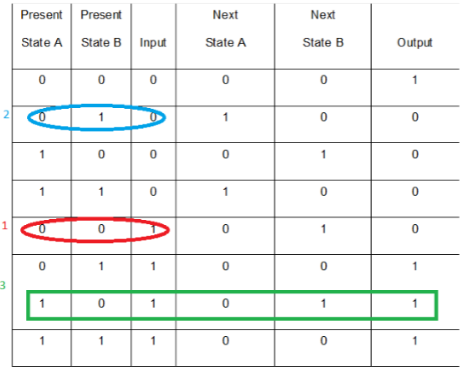QUESTION: 12

Which of the following statements is false?

Solution:
• A language is recursively enumerable if there exists a Turing machine that accepts every string of the language, and does not accept strings that are not in the language. Strings that are not in the language may be rejected or may cause the Turing machine to go into an infinite loop.
• A recursive language can't go into an infinite loop, it has to clearly reject the string, but a recursively enumerable language can go into an infinite loop.
• So, every recursive language is also recursively enumerable. Thus, the statement ‘Every subset of a recursively enumerable set is recursive’ is false.

Thus, option (C) is the answer.

QUESTION: 13

Given below are two finite state automata (→ indicates the start state and F indicates a final state)Which of the following represents the product automaton Z×Y?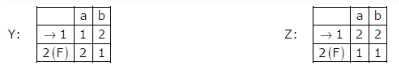Solution:

Solution for the problem is :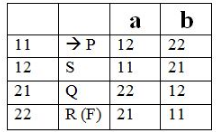Thus, option (A) is correct.

Please comment below if you find anything wrong in the above post.

QUESTION: 14

Match the following NFAs with the regular expressions they correspond to:

1. ϵ + 0(01*1 + 00) * 01*
2. ϵ + 0(10 *1 + 00) * 0
3. ϵ + 0(10 *1 + 10) *1
4. ϵ + 0(10 *1 + 10) *10 *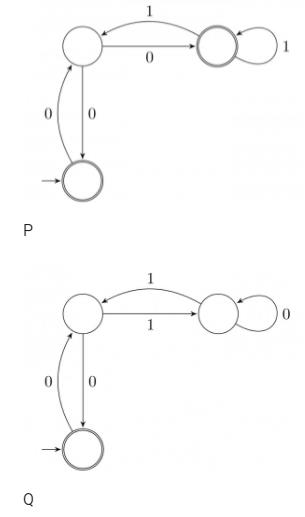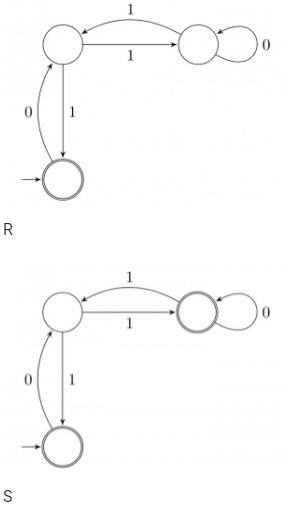Solution:

Trick: Here we see in all the given figures then second state has a loop over the input alphabets. In such cases we should resolve the loop at that state and transform the NFA into a simpler one to get a regular expression for the NFA. For resolving the loop, first reach the state where loop is to be resolved then draw all loops over that state and all possible movements to move to the final state.

Figure P: (when loop resolved at middle state)

Loop at middle state is either by a 00 or a 01*1.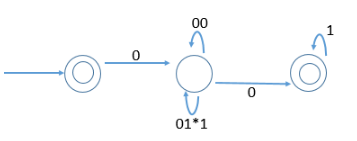Hence the regular expression: € + 0(00 +01*1)* 01*

Figure Q: (when loop resolved at middle state)

Loop at middle state is either by a 00 or a 10*1.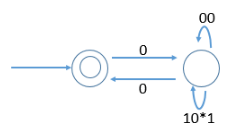Hence the regular expression: € + 0(00 +10*1)* 0.

Figure R: (when loop resolved at middle state)

Loop at middle state is either by a 10 or a 10*1.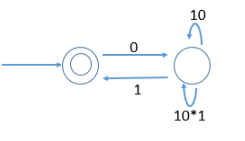Hence the regular expression: € + 0(10 +10*1)* 1.

Figure S: (when loop resolved at middle state)

Loop at middle state is either by a 10 or a 10*1.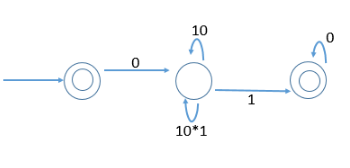Hence the regular expression: € + 0(10 +10*1)* 10*. The regular expressions matching with the above gives suitable matching as P-1, Q-2, R-3, S-4

QUESTION: 15

Which of the following are regular sets?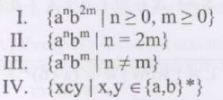Solution:

I. L = {a n b 2m |m, n ≥ 0} is a regular language of the form a ∗ (bb) ∗ .
II. L = {a n b m |n = 2m} is not a regular language. This is a similar language as of {a n b n} which is known to be not a regular language.
III. L = {a n b m |n notEqualTo m} is not regular because there is no way to for finite automaton to remember n, number of as.
IV. L = {xcy|x, y ∈ {a, b} ∗} is a regular language which is concatenation of {x|x ∈ a, b ∗ } on itself with a alphabet c in between.
Note: Regular languages are closed under concatenation. Hence, correct answer would be (A) I and IV only.

QUESTION: 16

Which of the following is TRUE?

Solution:

Some points for Regular Sets:

• A set is always regular if it is finite.
• A set is always regular if a DFA/NFA can be drawn for it.

Option A: Every subset of a regular set is regular is False. For input alphabets a and b, a*b* is regular. A DFA can be drawn for a*b* but a n b n for n≥0 which is a subset of a*b* is not regular as we cannot define a DFA for it.
Option B: Every finite subset of a non-regular set is regular is True. Each and every set which is finite can have a well-defined DFA for it so whether it is a subset of a regular set or non-regular set it is always regular.
Option C: The union of two non-regular sets is not regular is False. For input alphabets a and b, an bn for all n≥0 is non-regular as well as an bm for n≠m is also non- regular but their union is a*b* which is regular.
Option D: TInfinite union of finite sets is regular is False. For input alphabets a and b sets {ab}, {aabb}, {aaabbb}…….. are regular but their union {ab} U {aabb} U {aaabbb} U …………………….. gives {a n b n for n>0} which is not regular.

QUESTION: 17

A minimum state deterministic finite automaton accepting the language L={w | w ε {0,1} *, number of 0s and 1s in w are divisible by 3 and 5, respectively} has

Solution:
• Here a string w of 0's and 1's should have the property that, the no of 0's in the string w should be divisible by 3 (N(0) % 3 =0), and the number of 1's the string w should be divisible by 5 (N(1) % 5 =0).
• Having said that, the Language will contain the strings such as {ε , 000, 11111, 00011111, 00111101 , 11111000, 10101011 , 00000011111,....and so on}
• So, strings accepted by the automaton have to be of length 0, 3, 5, 8, 11, 13, 14, 16....and so on, i.e. equation for length will be 3x + 5y (where x,y>=0) Modulo 3 gives remainder as (0, 1, 2) , and Modulo 5 gives remainder as (0, 1, 2, 3, 4).

Hence 3 * 5 states, i.e. there will be 15 states in the automaton to represent this.

QUESTION: 18

Which of the following languages is regular?

Solution:

(C) Strings which are the part of this language are either 0w0 or 1w1 where w is any string in {0, 1}*. Thus, the language given in option (C) is regular.

All other languages accept strings that have a palindrome as their substring.
(A) Strings intersect with 0*110* .
(B) Strings intersect with 0*110*1 .
(D) Strings intersect with 10*110* .
According to the pumping lemma, languages given options (A), (B) and (D) are irregular.
Thus, option (C) is the answer.

QUESTION: 19

Consider the following Finite State Automaton:The language accepted by this automaton is given by the regular expression

Solution:
• In this case, we would at least have to reach q1 so that our string gets accepted. So, b* a is the smallest accepted string.
• Now, at q1, any string with any number of a’s and b’s would be accepted. So, we append (a + b)* to the smallest accepted string.
• Thus, the string accepted by the FSA is b* a(a + b)*.

Thus, C is the correct choice.

QUESTION: 20

Consider the automata given in previous question. The minimum state automaton equivalent to the above FSA has the following number of states

Solution:

Following is equivalent FSA with 2 states.